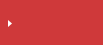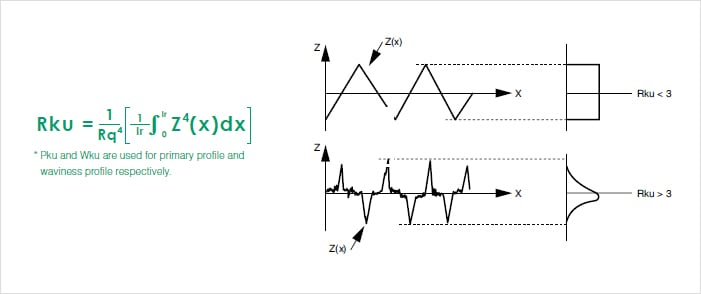>  What is line roughness?  >  Surface Roughness Parameters

# Surface Roughness Parameters

## Surface roughness parameters in JIS B 0601

• Peaks and valleys in the height direction
• Average amplitude in the height direction
• Average characteristics in the height direction
• Horizontal direction
• Hybrid
• Areal material ratio curve and probability density functionSearch from the parameters list

## Kurtosis (Rku, Pku, Wku)

Kurtosis uses the fourth power of the root mean square deviation to display the dimensionless fourth power of the sampling length Z(x).## Surface Roughness Parameters

This section explains the main parameters of ISO 4287:1997. Each parameter is classified according to primary profile (P), roughness profile (R), and waviness profile (W) in order to evaluate different aspects of the profile. (When the wavelengths of the waviness and primary profile components are compared, the surface roughness component is the asperity component of that which has the comparatively shorter wavelength.)## Search from the parameters list

Peaks and valleys in the height direction Arithmetical mean height (Ra, Pa, Wa) Maximum height of profile (Rz, Pz, Wz) Maximum profile peak height (Rp, Pp, Wp) Maximum profile valley depth (Rv, Pv, Wv) Mean height of profile elements (Rc, Pc, Wc) Total height of profile (Rt, Pt, Wt) Root mean square deviation (Rq, Pq, Wq) Skewness (Rsk, Psk, Wsk) Kurtosis (Rku, Pku, Wku) Mean width of the profile elements (RSm, PSm, WSm) Root mean square slope (RΔq, PΔq, WΔq) Load length ratio (Rmr (c), Pmr (c), Wmr (c)) Profile cut level difference (Rδc, Pδc, Wδc) Relative load length ratio (Rmr, Pmr, Wmr) Material ratio curve (BAC) Probability density function (ADF)